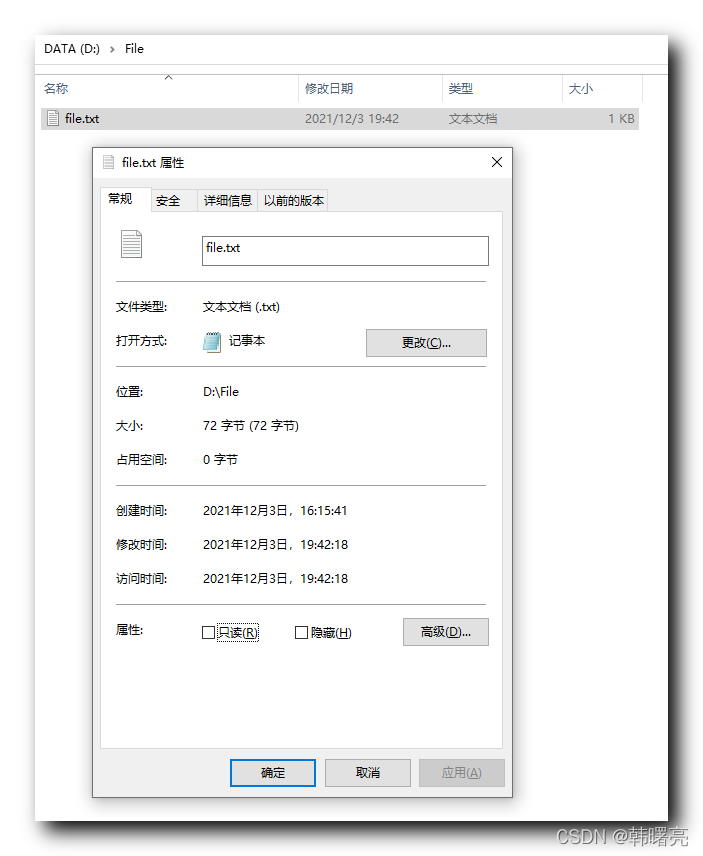# 高阶函数，文件

+关注继续查看

###############高阶函数#################

（1）函数本身也可以赋值给变量,即:变量可以指向函数。（2）函数名其实就是指向函数的变量!1.map函数

map()函数接收两个参数,一个是函数,一个是序列, map 将传入的函数依次作用到序列的每个元素,并把结果作为新的 list 返回。

(不需要 map() 函数,写一个循环,也可以计算出结果.map函数)2.reduce函数

reduce 把一个函数作用在一个序列[x1, x2, x3...]上,这个函数必须接收两个参数,reduce 把结果继续和序列的下一个元素做累积计算。reduce函数map/reduce练习题:

def fun(x): #####  定义一个函数，

return x.title()

li = ['adam', 'LISA', 'barT']  ###定义一个列表

print map(fun ,li)

Python 提供的 sum() 函数可以接受一个 list 并求和,请编写一个 prod()函数,可以接受一个 list 并利用 reduce() 求积。3.filter函数

filter() 也接收一个函数和一个序列。和 map() 不同的时,filter() 把传入的函数依次作用于每个元素,然后根据返回值是 True4.sorted函数

x < y, return -1

x == y, return 0

x > y, return 1

python内置的 sorted() 函数就可以对 list 进行排序;

In : t=(12,56,432,56,954)  ####定义一个元组

In : sorted(t)  #####内置函数 :默认从小到大排列

Out: [12, 56, 56, 432, 954]

In : def reversed_cmp(x,y): #####自己定义函数：从大到小排列。（整数）

if x>y :

...:         return -1

...:     elif x<y :

...:         return 1

...:     else:

...:         return 0

...:

In : sorted(t,reversed_cmp) ###高阶函数

Out: [954, 432, 56, 56, 12]

In : li=["xiaoming","huangrong"]  ####定义一个列表

In : sorted(li)  #####内置函数 :默认从小到大排列，按字母所对应的acsii码大小排列。

Out: ['huangrong', 'xiaoming']

In : li=['xiaoming', 'huangrong', 'Huangrong']

In : "huangrong">"Huangrong"

Out: True

In : def ignore_case_cmp(x,y):#####自己定义函数：从大到小排列（字母）

lower1=x.lower()

lower2=y.lower()

if lower1<lower2:

return -1

elif lower1>lower2:

....:         return 1

....:     else:

....:         return 0

....:

In : sorted(li,ignore_case_cmp)  ###高阶函数

Out: ['huangrong', 'Huangrong', 'xiaoming']

5.函数作为返回值

（1）

def lazy_sum(*args):    1

def sum():              5

ax = 0                  6

for n in args:          7

ax = ax + n             8

return ax               9

return sum              3

f = lazy_sum(1,2,3,4)   2    #调用 lazy_sum() 时,返回的并不是求和结果,而是求和函数

f()                    4,10 #调用函数 f 时,才真正计算求和的结果函数作为返回值调用 lazy_sum() 时,每次调用都会返回一个新的函数,即使传入相同的参数

（2）

def warp_sum(*args):定义一个把求和函数作为内置函数的函数：执行显示表达式（闭包）

def my_sum():  ########定义一个求和的函数：执行直接求和

sum_num=0

for i in args:

if not isinstance(i,(int,float)):

print "type error"

sum_num = sum_num +i

return sum_num

return my_sum

f = warp_sum(1,4,2)   ####给函数赋值

print f()   #######打印f（3）

def count(): #########定义一个函数

fs=[]   ######定义一个空列表

for i in range(1,4):  ###循环遍历

def f():

return i*i

fs.append(f)  ####将f添加到fs列表，f是函数的存储地址

return fs

f1,f2,f3,=count()

print f1  ########显示函数的存储地址

print f2

print f3（4）

def count():

fs=[]

for i in range(1,4):

def f():

return i*i

fs.append(f)

return fs

f1,f2,f3,=count()

print f1()  #############显示的是数值，可是数值都为9

print f2()

print f3()（5）

def count():

fs=[]

for i in range(1,4):

def f(j):

def g():##########在f函数里嵌套一个g函数

return j*j

return g

fs.append(f(i))

return fs

f1,f2,f3,=count()

print f1()   ####返回的是各自对应值；1，4，9

print f2()

print f3()6.匿名函数

此外,匿名函数也是一个函数对象,也可以把匿名函数赋值给一个变量,再利用变量来调用该函数;

def pow1(x):

return x*x

print map(pow1,range(1,11))

print map(lambda x:x*x,range(1,11))

f= lambda :1

print ff=lambda x,y=2:x**y

print f(2,3)

print (2)f = lambda  *x:map(lambda  x:x+x,x)

print f(1,2,3,4)f = lambda  **kwargs:kwargs.items()

print f (name='fentiao',age=5)x=1

oper = "*"

y = 2

d={

"+":lambda x,y:x+y,

"-":lambda x,y:x+y,

"*":lambda x,y:x*y,

"/":lambda x,y:x/y

}

if oper not in d.keys():

print 'input +,-,*,/'7 装饰器

import time

def timeIt(func):

def warp(arg):

start = datetime.datetime.now()

func(arg)

end = datetime.datetime.now()

cost = end - start

print "execute %s spend %s" % (func.__name__,cost.total_seconds())

return warp

@timeIt # 这里是 python 提供的一个语法糖

def func(arg):

time.sleep(arg)

func(3)

import time

def timmer (func):

start_time = time.time()

func()

stop_time=time.time()

return stop_time-start_time

def hello2():

print 'hello2....'

time.sleep(2)

print timmer(hello2)

print d[oper](x,y)import time

def logger (func):

def dec():

start_time = time.time()

func()

stop_time=time.time()

return '%s run %f s'%(func.__name__,stop_time-start_time)

return dec

@logger

def hello2():

print 'hello2....'

time.sleep(2)

print hello2()

#############文件操作#################

Python 内置了读写文件的函数,用法和 C 是兼容的。

1.思考:

2.思考文件读写的过程:

（1）. 打开文件

（2）. 向文件中写入内容;

（3）. 关闭文件文件读写

3. 打开文件

f = open('/root/hello')

# 如果文件不存在, open() 函数就会抛出一个 IOError 的错误,并且给出错误码和详细的信息告诉你文件不存在;

4.读取文件

5.关闭文件

f.close()

#文件使用完毕后必须关闭,因为文件对象会占用操作系统的资源。

6.思考:7. 二进制文件

>>> f = open('/root/test.jpg', 'rb')

'\xff\xd8\xff\xe1\x00\x18Exif\x00\x00...' # 十六进制表示的字节文件读写

8. 字符编码

import codecs

with codecs.open('/Users/michael/gbk.txt', 'r', 'gbk') as f:

r 以读的方式打开,定位到文件开头 , 默认的 mode

r+ 以读写的方式打开,定位文件开头 , 可以写入内容到文件

w 以写的方式打开,打开文件的时候会清空文件的内容,并且不能读

w+ 以读写的方式打开,定位到文件头,并且打开文件的时候也会清空文件的内容

a 以写的方式打开,定位到文件的末尾,是一个追加的操作 , 但并不允许读

a+ 以读写的方式打开,定位到文件的末尾,追加的方式。1.f.flush()函数,将缓冲区的内容写入到硬盘中

2.f.seek(offset[,whence]),offset 表示移动多少字节, whence 为 1 的时候表示相对于当前位置移动的;当 2 的时候从文件的末尾往后移动,但不一定所有的平台都支持;默认为 0 表示从文件开头往后移动

3.f.tell()函数,返回当前文件指针的偏移量:文件的其它操作

4.fileno() 函数,返回当前的文件描述符,一个数字

5.isatty() 函数,当前打开的文件是否是一个终端设备

6.closed 属性,当前文件是否关闭 ,|True,False, f.closed

7.file 对象是一个迭代器:

next() 方法 , 一行一行的读 , 每次读取一行with expression as obj:# 将打开的对象赋值给 obj

expression

#obj 的作用域只在 with 语句中660 0《MATLAB图像处理超级学习手册》一一2.8 M文件与M函数
2425 0php 排查函数文件执行路径的打印
889 0698 020122 0PHP文件下载函数(从MySQL提取文件路径)
<?php header("Content-type: text/html;charset=utf-8"); require("include/MysqlConn.php"); // 获得ID $id=$_GET['id']; // 判断是否有参数 if (!\$id) {     die ("缺少.
2593 0713 023538 0Linux 文件操作函数
1312 0【C 语言】文件操作 ( 按照内存块的方式读写文件 | fread 函数 | fwrite 函数 )
【C 语言】文件操作 ( 按照内存块的方式读写文件 | fread 函数 | fwrite 函数 )
41 0
20380

0

JS零基础入门教程（上册）